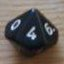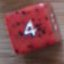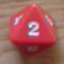TROLL: about rerolling and not-number values

## TROLL: about rerolling and not-number values

 Mon Aug 18 20:31:24 2014   by   Tiziano Contorno You have a d6 with special faces: one face is "A", 2 faces are "B", 3 faces are "C". If we could use a collection of letters it would be:choose{A,B,B,C,C,C}You roll N of these dice, the result is the count of the same type of face (sometimes I'm interested in "A", sometimes in "B", sometimes in "C").You get a reroll (you can keep as many dice as you wish, reroll the remaining and keep the result): obviously when I calculate probability of "A", in the first roll I will keep all the "A" and reroll only the dice with face different from "A", same logic applies for "B" and "C". I'm trying to calculate the probability of doing how many "A", "B" or "C". I assigned an integer to each type of face (A=2, B=1, C=0).For instance, I count the number of "A" in a N dice roll:count 2 = N# choose{2,1,1,0,0,0}My logic for the two rolls is (assuming I'm looking for "A" = 2):FIRSTROLL := N# choose{2,1,1,0,0,0}GOODDICE := count 2 = FIRSTROLLSECONDROLL := count 2 = (N - GOODDICE)# choose{2,1,1,0,0,0}FINALCOUNT := FIRSTROLL + SECONDROLLBut I'm stuck and I don't know how to make it happen.Thank you for your help. Tue Aug 19 14:24:10 2014   by   Torben Your idea is sound, you just have a few syntactic errors (variable names can't use underscores, for example).  This should work:`N := 5; \ dice pool sizeT := 2; \ target numberFirstRoll := count T = N # choose{2,1,1,0,0,0};SecondRoll := count T = (N - FirstRoll) # choose{2,1,1,0,0,0};FirstRoll + SecondRoll` Tue Aug 19 15:17:18 2014   by   Tiziano Contorno Thank you Torben! It works perfectly.I can now play with multiple rerolls, ie 2 rerolls would be:N := 6; \ dice pool sizeT := 2; \ target numberFirstRoll := count T = N # choose{2,1,1,0,0,0};SecondRoll := count T = (N - FirstRoll) # choose{2,1,1,0,0,0};ThirdRoll := count T = (N - FirstRoll - SecondRoll) # choose{2,1,1,0,0,0};FirstRoll + SecondRoll + ThirdRollI think I can use this knowledge to post King of Tokyo dice dynamics.